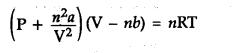# Explain the physical significance of van der Waal's parameters

In the reactionwhere V and ‘b’ are the van der Walls parameters which depend upon nature of gas.
Significance of constant ‘V : The constant 'V is excluded volume per mol of a gas.
Its units are L {{mol}^{-1}} The volume of ‘V is four times the actual volume of the molecules.
Significance of constant ‘a’ : It gives the ideal of magnitude of attractive forces between the gas molecules. Its units are atm {{L}^{2}} {{mol}^{-2}}. Larger the value of ‘a’ Larger will be the intermolecular attraction among the gas molecules.